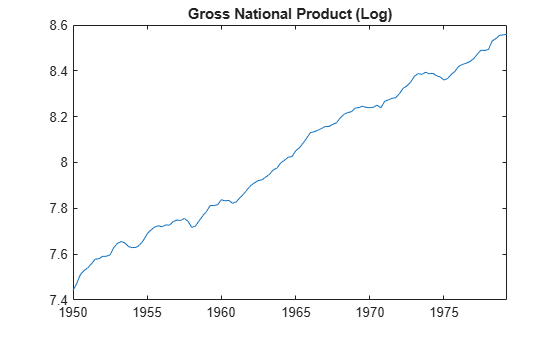# Use Hodrick-Prescott Filter to Reproduce Original Result

This example shows how to use the Hodrick-Prescott filter to decompose a time series.

The Hodrick-Prescott filter separates a time series into growth and cyclical components with

${y}_{t}={g}_{t}+{c}_{t}$

where ${\mathit{y}}_{\mathit{t}}$ is a time series, ${\mathit{g}}_{\mathit{t}}$ is the growth component of ${\mathit{y}}_{\mathit{t}}$, and ${\mathit{c}}_{\mathit{t}}$ is the cyclical component of ${\mathit{y}}_{\mathit{t}}$ for $\mathit{t}=1,...,\mathit{T}$.

The objective function for the Hodrick-Prescott filter has the form

$\sum _{t=1}^{T}{c}_{t}^{2}+\lambda \sum _{t=2}^{T-1}\left(\left({g}_{t+1}-{g}_{t}\right)-\left({g}_{t}-{g}_{t-1}\right){\right)}^{2}$

with a smoothing parameter $\lambda$. The programming problem is to minimize the objective over all ${\mathit{g}}_{1},...,{\mathit{g}}_{\mathit{T}}$.

The conceptual basis for this programming problem is that the first sum minimizes the difference between the data and its growth component (which is the cyclical component) and the second sum minimizes the second-order difference of the growth component, which is analogous to minimization of the second derivative of the growth component.

Note that this filter is equivalent to a cubic spline smoother.

### Use Hodrick-Prescott Filter to Analyze GNP Cyclicality

Using data similar to the data found in Hodrick and Prescott , plot the cyclical component of GNP. This result should coincide with the results in the paper. However, since the GNP data here and in the paper are both adjusted for seasonal variations with conversion from nominal to real values, differences can be expected due to differences in the sources for the pair of adjustments. Note that our data comes from the St. Louis Federal Reserve FRED database , which was downloaded with the Datafeed Toolbox™.

load Data_GNP % Change the following two lines to try alternative periods startdate = datetime(1950,1,1); enddate = datetime(1979,4,1); DTT = DataTimeTable(startdate:enddate,:); DTT.GNPRLog = log(DTT.GNPR);

We run the filter for different smoothing parameters $\lambda$ = 400, 1600, 6400, and $\infty$. The infinite smoothing parameter just detrends the data.

[TTbl4,CTbl4] = hpfilter(DTT,400,DataVariables="GNPRLog"); [TTbl16,CTbl16] = hpfilter(DTT,1600,DataVariables="GNPRLog"); [TTbl64,CTbl64] = hpfilter(DTT,6400,DataVariables="GNPRLog"); [TTblInf,CTblInf] = hpfilter(DTT,Inf,DataVariables="GNPRLog");

### Plot Cyclical GNP and Its Relationship With Long-Term Trend

The following code generates Figure 1 from Hodrick and Prescott .

plot(DTT.Time,CTbl16.GNPRLog,"b"); hold all plot(DTT.Time,CTblInf.GNPRLog - CTbl16.GNPRLog,"r"); title("\bf Figure 1 from Hodrick and Prescott"); ylabel("\bf GNP Growth"); legend(["Cyclical GNP" "Difference"]); hold offThe blue line is the cyclical component with smoothing parameter 1600 and the red line is the difference with respect to the detrended cyclical component. The difference is smooth enough to suggest that the choice of smoothing parameter is appropriate.

### Statistical Tests on Cyclical GNP

We will now reconstruct Table 1 from Hodrick and Prescott . With the cyclical components, we compute standard deviations, autocorrelations for lags 1 to 10, and perform a Dickey-Fuller unit root test to assess non-stationarity. As in the article, we see that as lambda increases, standard deviations increase, autocorrelations increase over longer lags, and the unit root hypothesis is rejected for all but the detrended case. Together, these results imply that any of the cyclical series with finite smoothing is effectively stationary.

ACFTbl4 = autocorr(CTbl4,NumLags=10,DataVariable="GNPRLog"); ACFTbl16 = autocorr(CTbl16,NumLags=10,DataVariable="GNPRLog"); ACFTbl64 = autocorr(CTbl64,NumLags=10,DataVariable="GNPRLog"); ACFTblInf = autocorr(CTblInf,NumLags=10,DataVariable="GNPRLog"); [StatTbl4] = adftest(CTbl4,Model="ARD"); [StatTbl16] = adftest(CTbl16,Model="ARD"); [StatTbl64] = adftest(CTbl64,Model="ARD"); [StatTblInf] = adftest(CTblInf,Model="ARD"); displayResults(CTbl4,CTbl16,CTbl64,CTblInf, ... ACFTbl4,ACFTbl16,ACFTbl64,ACFTblInf, ... StatTbl4,StatTbl16,StatTbl64,StatTblInf);
Table 1 from Hodrick and Prescott Reference Smoothing Parameter 400 1600 6400 Infinity Std. Dev. 1.52 1.75 2.06 3.11 Autocorrelations 1 0.74 0.78 0.82 0.92 2 0.38 0.47 0.57 0.81 3 0.05 0.17 0.33 0.70 4 -0.21 -0.07 0.12 0.59 5 -0.36 -0.24 -0.03 0.50 6 -0.39 -0.30 -0.10 0.44 7 -0.35 -0.31 -0.13 0.39 8 -0.28 -0.29 -0.15 0.35 9 -0.22 -0.26 -0.15 0.31 10 -0.19 -0.25 -0.17 0.26 Unit Root -4.35 -4.13 -3.79 -2.28 Reject H0 1 1 1 0 

### Local Function

function displayResults(gnpcycle4,gnpcycle16,gnpcycle64,gnpcycleinf,... gnpacf4,gnpacf16,gnpacf64,gnpacfinf,... gnptest4,gnptest16,gnptest64,gnptestinf) % DISPLAYRESULTS Display cyclical GNP test results in tabular form fprintf(1,'Table 1 from Hodrick and Prescott Reference\n'); fprintf(1,' %10s %s\n',' ','Smoothing Parameter'); fprintf(1,' %10s %10s %10s %10s %10s\n',' ','400','1600','6400','Infinity'); fprintf(1,' %-10s %10.2f %10.2f %10.2f %10.2f\n','Std. Dev.', ... 100*std(gnpcycle4.GNPRLog),100*std(gnpcycle16.GNPRLog), ... 100*std(gnpcycle64.GNPRLog),100*std(gnpcycleinf.GNPRLog)); fprintf(1,' Autocorrelations\n'); for i = 2:11 fprintf(1,' %10g %10.2f %10.2f %10.2f %10.2f\n',(i-1), ... gnpacf4.ACF(i),gnpacf16.ACF(i),gnpacf64.ACF(i),gnpacfinf.ACF(i)) end fprintf(1,' %-10s %10.2f %10.2f %10.2f %10.2f\n','Unit Root', ... gnptest4.stat,gnptest16.stat,gnptest64.stat,gnptestinf.stat); fprintf(1,' %-10s %10d %10d %10d %10d\n','Reject H0',... gnptest4.h,gnptest16.h,gnptest64.h,gnptestinf.h); end

 Hodrick, Robert J, and Edward C. Prescott. "Postwar U.S. Business Cycles: An Empirical Investigation." Journal of Money, Credit, and Banking. Vol. 29, No. 1, 1997, pp. 1–16.

 U.S. Federal Reserve Economic Data (FRED), Federal Reserve Bank of St. Louis, https://fred.stlouisfed.org/.